Anzeige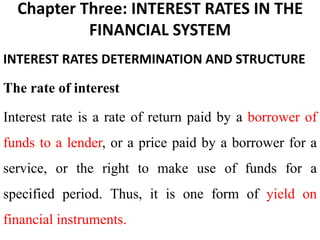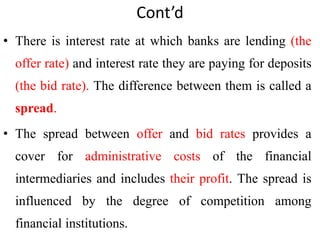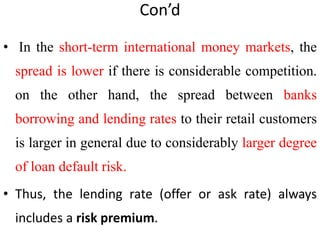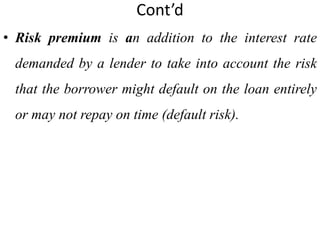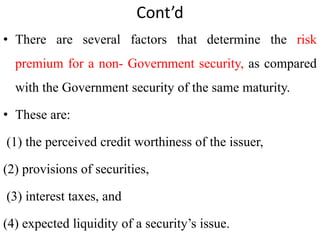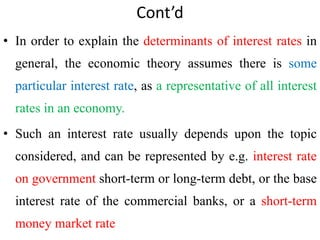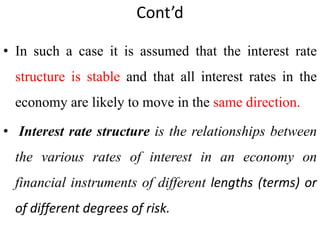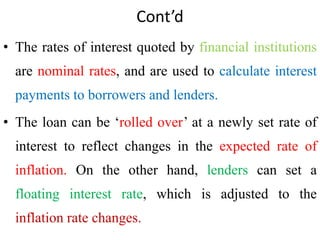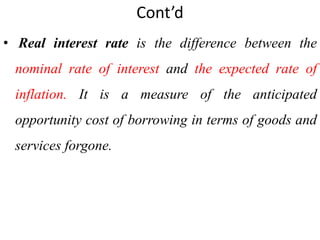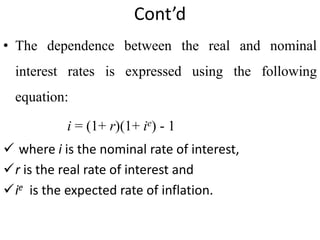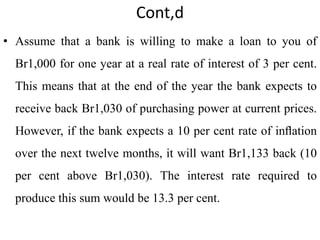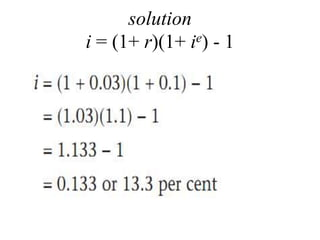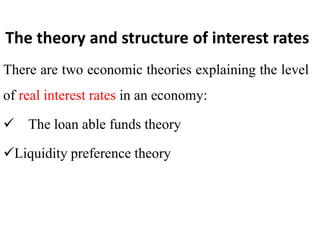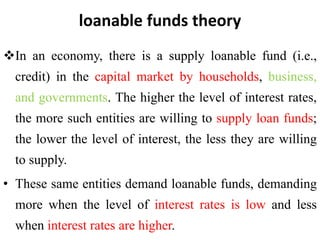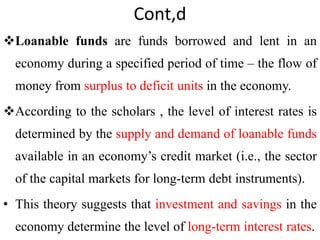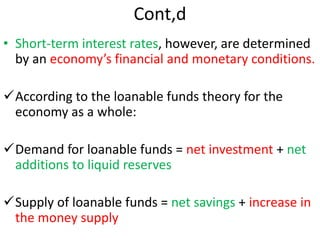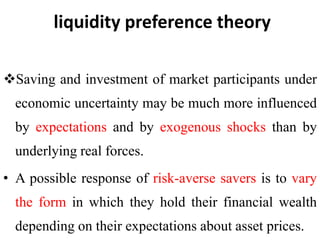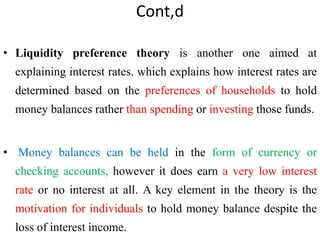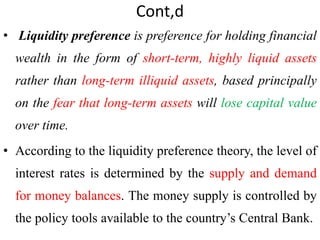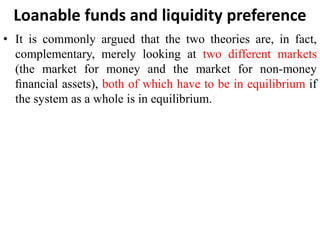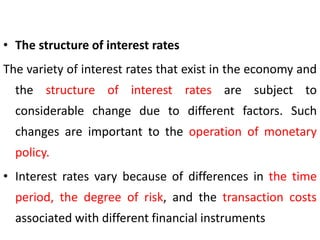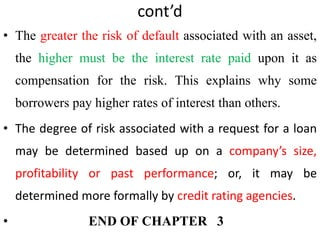1 von 22
Anzeige

### chapter three interest rates in the financial system.pptx

1. Chapter Three: INTEREST RATES IN THE FINANCIAL SYSTEM INTEREST RATES DETERMINATION AND STRUCTURE The rate of interest Interest rate is a rate of return paid by a borrower of funds to a lender, or a price paid by a borrower for a service, or the right to make use of funds for a specified period. Thus, it is one form of yield on financial instruments.
2. Cont’d • There is interest rate at which banks are lending (the offer rate) and interest rate they are paying for deposits (the bid rate). The difference between them is called a spread. • The spread between offer and bid rates provides a cover for administrative costs of the financial intermediaries and includes their profit. The spread is influenced by the degree of competition among financial institutions.
3. Con’d • In the short-term international money markets, the spread is lower if there is considerable competition. on the other hand, the spread between banks borrowing and lending rates to their retail customers is larger in general due to considerably larger degree of loan default risk. • Thus, the lending rate (offer or ask rate) always includes a risk premium.
4. Cont’d • Risk premium is an addition to the interest rate demanded by a lender to take into account the risk that the borrower might default on the loan entirely or may not repay on time (default risk).
5. Cont’d • There are several factors that determine the risk premium for a non- Government security, as compared with the Government security of the same maturity. • These are: (1) the perceived credit worthiness of the issuer, (2) provisions of securities, (3) interest taxes, and (4) expected liquidity of a security’s issue.
6. Cont’d • In order to explain the determinants of interest rates in general, the economic theory assumes there is some particular interest rate, as a representative of all interest rates in an economy. • Such an interest rate usually depends upon the topic considered, and can be represented by e.g. interest rate on government short-term or long-term debt, or the base interest rate of the commercial banks, or a short-term money market rate
7. Cont’d • In such a case it is assumed that the interest rate structure is stable and that all interest rates in the economy are likely to move in the same direction. • Interest rate structure is the relationships between the various rates of interest in an economy on financial instruments of different lengths (terms) or of different degrees of risk.
8. Cont’d • The rates of interest quoted by financial institutions are nominal rates, and are used to calculate interest payments to borrowers and lenders. • The loan can be ‘rolled over’ at a newly set rate of interest to reflect changes in the expected rate of inflation. On the other hand, lenders can set a floating interest rate, which is adjusted to the inflation rate changes.
9. Cont’d • Real interest rate is the difference between the nominal rate of interest and the expected rate of inflation. It is a measure of the anticipated opportunity cost of borrowing in terms of goods and services forgone.
10. Cont’d • The dependence between the real and nominal interest rates is expressed using the following equation: i = (1+ r)(1+ ie) - 1  where i is the nominal rate of interest, r is the real rate of interest and ie is the expected rate of inflation.
11. Cont,d • Assume that a bank is willing to make a loan to you of Br1,000 for one year at a real rate of interest of 3 per cent. This means that at the end of the year the bank expects to receive back Br1,030 of purchasing power at current prices. However, if the bank expects a 10 per cent rate of inﬂation over the next twelve months, it will want Br1,133 back (10 per cent above Br1,030). The interest rate required to produce this sum would be 13.3 per cent.
12. solution i = (1+ r)(1+ ie) - 1
13. The theory and structure of interest rates There are two economic theories explaining the level of real interest rates in an economy:  The loan able funds theory Liquidity preference theory
14. loanable funds theory In an economy, there is a supply loanable fund (i.e., credit) in the capital market by households, business, and governments. The higher the level of interest rates, the more such entities are willing to supply loan funds; the lower the level of interest, the less they are willing to supply. • These same entities demand loanable funds, demanding more when the level of interest rates is low and less when interest rates are higher.
15. Cont,d Loanable funds are funds borrowed and lent in an economy during a specified period of time – the flow of money from surplus to deficit units in the economy. According to the scholars , the level of interest rates is determined by the supply and demand of loanable funds available in an economy’s credit market (i.e., the sector of the capital markets for long-term debt instruments). • This theory suggests that investment and savings in the economy determine the level of long-term interest rates.
16. Cont,d • Short-term interest rates, however, are determined by an economy’s financial and monetary conditions. According to the loanable funds theory for the economy as a whole: Demand for loanable funds = net investment + net additions to liquid reserves Supply of loanable funds = net savings + increase in the money supply
17. liquidity preference theory Saving and investment of market participants under economic uncertainty may be much more influenced by expectations and by exogenous shocks than by underlying real forces. • A possible response of risk-averse savers is to vary the form in which they hold their financial wealth depending on their expectations about asset prices.
18. Cont,d • Liquidity preference theory is another one aimed at explaining interest rates. which explains how interest rates are determined based on the preferences of households to hold money balances rather than spending or investing those funds. • Money balances can be held in the form of currency or checking accounts, however it does earn a very low interest rate or no interest at all. A key element in the theory is the motivation for individuals to hold money balance despite the loss of interest income.
19. Cont,d • Liquidity preference is preference for holding financial wealth in the form of short-term, highly liquid assets rather than long-term illiquid assets, based principally on the fear that long-term assets will lose capital value over time. • According to the liquidity preference theory, the level of interest rates is determined by the supply and demand for money balances. The money supply is controlled by the policy tools available to the country’s Central Bank.
20. Loanable funds and liquidity preference • It is commonly argued that the two theories are, in fact, complementary, merely looking at two different markets (the market for money and the market for non-money ﬁnancial assets), both of which have to be in equilibrium if the system as a whole is in equilibrium.
21. • The structure of interest rates The variety of interest rates that exist in the economy and the structure of interest rates are subject to considerable change due to different factors. Such changes are important to the operation of monetary policy. • Interest rates vary because of differences in the time period, the degree of risk, and the transaction costs associated with different financial instruments
22. cont’d • The greater the risk of default associated with an asset, the higher must be the interest rate paid upon it as compensation for the risk. This explains why some borrowers pay higher rates of interest than others. • The degree of risk associated with a request for a loan may be determined based up on a company’s size, profitability or past performance; or, it may be determined more formally by credit rating agencies. • END OF CHAPTER 3
Anzeige